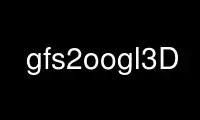# gfs2oogl3D - Online in the CloudThis is the command gfs2oogl3D that can be run in the OnWorks free hosting provider using one of our multiple free online workstations such as Ubuntu Online, Fedora Online, Windows online emulator or MAC OS online emulator

### PROGRAM:

#### NAME

gfs2oogl2D, gfs2oogl3D - converts a Gerris simulation file to other (graphical) formats.

#### SYNOPSIS

gfs2oogl [OPTION]<GFS_FILE

#### DESCRIPTION

This manual page documents briefly the gfs2oogl command.

#### OPTIONS

These programs follow the usual GNU command line syntax, with long options starting with
two dashes (`-'). A summary of options is included below.

-u V, --iso=V
Outputs a GTS file isosurface for value V the variable needs to be specified using
-c.

-f D, --stream=D
Draw evenly-spaced streamlines (D is the spacing).

-I M, --dmin=M
Controls length of evenly-spaced streamlines default is 0.5.

-O M, --maxcost=M
Controls compression of streamlines (default is 2e-7).

-b x,.., --box=x,y,..
Specify bounding box for streamline calculation.

-j, --closed
Outputs only closed streamlines.

-p F, --profile=F
Output list of values for coordinates defined in F.

-o, --mixed
Output text values in mixed cells only.

-L L, --level=L
Use cells at level L only.

-i, --reinit
Reinitializes refinement and solid fractions.

-e, --merged
Draw boundaries of merged cells.

-S, --squares
Draw (colored) squares.

-g, --gnuplot
Output gnuplot data.

-x VAL, --sx=VAL
outputs a GTS surface, cross section for x = VAL of the scalar variable.

-y VAL, --sy=VAL
outputs a GTS surface, cross section for y = VAL of the scalar variable.

-z VAL, --sz=VAL
outputs a GTS surface, cross section for z = VAL of the scalar variable.

-s S, --surface=S
outputs the surface defined by file S (or the solid surface is S is equal to
`solid').

-V S, --vector=S
output an OOGL representation of the velocity vector field in the mixed cells.

-l F, --streamlines=F
draw streamlines starting from each point defined in file F.

-C F, --cylinder=F
draw stream cylinders starting from each point defined in file F.

-R F, --ribbon=F
draw stream ribbons starting from each point defined in file F.

-r refines the solid surface according to the local resolution.

-c V, --color=V
color surfaces, streamlines etc... according to the.

-m V, --min=V
set minimum scalar value to V.

-M V, --max=V
set maximum scalar value to V.

-v, --verbose
display statistics and other info.

-h, --help
display the help and exit.

Use gfs2oogl3D online using onworks.net services